## ANOVA

Analysis of Variance.'' A Statistical Test for heterogeneity of Means by analysis of group Variances. To apply the test, assume random sampling of a variatewith equal Variances, independent errors, and a Normal Distribution. Letbe the number of Replicates (sets of identical observations) within each ofFactor Levels (treatment groups), and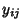be the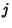th observation within Factor Level. Also assume that the ANOVA is balanced'' by restrictingto be the same for each Factor Level.

Now define the sum of square terms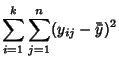(1)(2)(3)(4)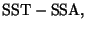(5)

which are the total, treatment, and error sums of squares. Here,is the mean of observations within Factor Level, and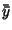is the group'' mean (i.e., mean of means). Compute the entries in the following table, obtaining the P-Value corresponding to the calculated F-Ratio of the mean squared values(6)

 Category SS °Freedom Mean Squared F-Ratio Treatment SSA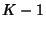Error SSETotal SST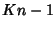If the P-Value is small, reject the Null Hypothesis that all Means are the same for the different groups.